SCHOLASTIC APTITUDE TEST - 1995.

MATHEMATICS

 Time: Two Hours Max. Marks: 60 (8.30 AM - 10.30 AM)

Answers must be written either in English or the medium of instruction of the candidate in high school.

• There will be no negative marking.
• There are FIVE parts.
• Answer all questions of a part at one place.
• Use of calculators or graph papers is not permitted.

PART A

This part contains five statements. State whether the statements are true or false. Give reasons. (5 x 1 = 5 Marks)

1. The product of three positive integers is equal to the product of their L.C.M and G.C.D.
2. 443 is prime number
3. (a – b)x2 +(b – c)x +(c – a) = 0 and (c – a)x2 +(b – c)x +(a – b) = 0 have a common solution for all real numbers a,b,c.
4. Every odd number can be expressed as a difference of two squares.
5. The graph of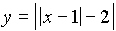cuts the x-axis exactly once.

PART B

This part contains FIVE questions. Each question has only one correct answer. Indicate your choice by marking with the correct letter. Show your working in brief. (5 x 2 = 10 Marks)

1. If a2+b2+c2 = D where a and b are consecutive positive integers and c = ab, then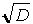is
(A) always an even integer
(B) sometimes an odd integer and sometimes not
(C) always an odd integer
(D) sometimes rational, sometimes not
(E) always irrational
2. A triangle ABC is to be constructed. Given side a ( opposite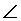A ),B and hc , the altitude drawn from C on to AB. If N is the number of non-congruent triangles then N is
(A) one   (B) two     (C) zero   (D) zero or infinite   (E) infinite
3. In any triangle ABC, a and b are the sides of triangle. Given that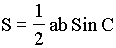( where S is the area of the triangle), then
 A)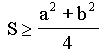B)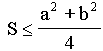C)D)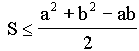E) None of these
4. If a0, a1, .............., a50 are respectively the coefficients of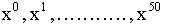in the expansion of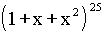, then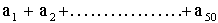is
 A) odd B) even C) Zero D) None of these
5. The smallest value of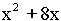for all real values of x is
 (A) –16.25 (B) –16 (C) –15 (D) –8 (E) None of these

PART C

This part contains FIVE incomplete statements. Complete the statements by filling the blanks. Write only the answers in your answer sheet strictly in the order in which the questions appear. (5 x 2 = 10 Marks)

1. The value of aÎ R for which the equation (1+a2)x2 +2(x-a)(1+ax)+1 = 0 has no real roots is _______________________________.
2. The greater of the two numbers 3114 and 1718 is _______________.
3. A and B are known verticies of a triangle. C is the unknown vertex. P is a known point in the plane of the triangle ABC. Of the two following hypotheses,
Hypothesis I - P is the incentre of D ABC
Hypothesis II - P is the circumcentre of D ABC.
The hypothesis which will enable C to be uniquely determined is ____I / II____ (Strike off the inapplicable)

4. If x = 0.101001000100001 ................ is the decimal expansion of positive x, and in the series expansion of x the first, second and third terms are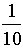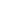,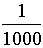, and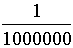then the 100 th term is _______________________.
5. If ABC is a triangle with AB = 7, BC = 9 and CA = n where n is a positive integer, then possible two values of n are ____________________.

PART D

 Attempt any FIVE questions. (5 x 5 = 25 Marks)
1. Solve the equations
xy + yz + zx = 12-x2  = 15-y2 = 20-z2
2. A is 2 x 2 matrix such that AB = BA whenever B is a 2 x 2 matrix. Prove that A must be of the form kI, where k is a real number and I the identity matrix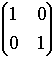.
3. Determine all 2 x 2 matrices A such that A2=I, where I =.
4. Find an explicit formula ( in terms of nÎ N ) for f(n), if f(1)=1 and f(n)=f(n –1) + 2n – 1
5. Let ABCD be a square. Let P,Q,R,S be respectively points on AB,BC,CD,DA such that PR and QS intersect at right angles. Show that PR=QS.
6. Let ABC be any triangle. Construct parallelograms ABDE and ACFG on the outside D ABC. Let P be any point where the lines DE and FG intersect. Construct a parallelogram BCHI on the outside D ABC such that PA and BI are equal and parallel. Show that Area of ABDE + Area of ACFG = Area of BCHI.
7. A belt wraps around two pulleys which are mounted with their centres s  apart. If the radius of one pulley is R and the radius of the other is (R+r)  , show that the length of the belt is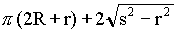.

PART E

 Attempt any ONE question (1 x 10 = 10 Marks)
1. Deduce pythogaras theorem from the result of problem 6 of part D.
2. Let x be any real number. Let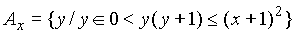and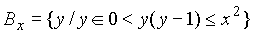. Prove that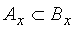.
3. Let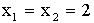. Show that there exists an infinite sequence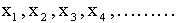such that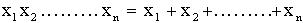for all positive integers n.